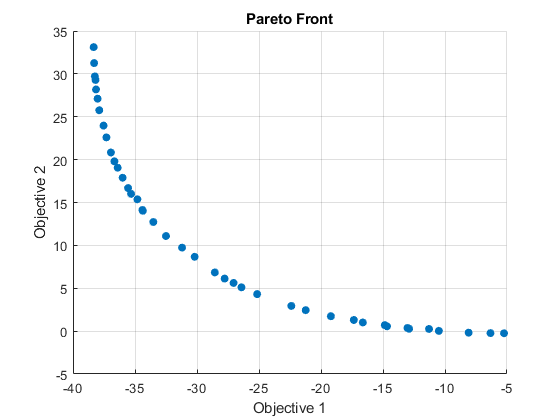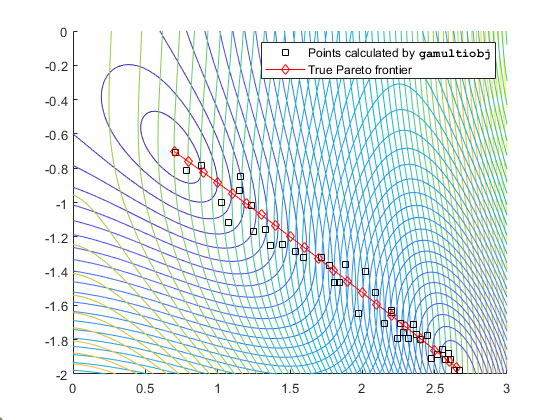Documentation

## Pareto Front for Two Objectives

### Multiobjective Optimization with Two Objectives

This example has a two-objective fitness function f(x), where x is also two-dimensional:

```function f = mymulti1(x) f(1) = x(1)^4 - 10*x(1)^2+x(1)*x(2) + x(2)^4 -(x(1)^2)*(x(2)^2); f(2) = x(2)^4 - (x(1)^2)*(x(2)^2) + x(1)^4 + x(1)*x(2);```

Create this function file before proceeding, and store it as `mymulti1.m` on your MATLAB® path.

### Performing the Optimization with Optimization App

1. To define the optimization problem, start the Optimization app, and set it as pictured.2. Set the options for the problem as pictured.3. Run the optimization by clicking under Run solver and view results.

A plot appears in a figure window.This plot shows the tradeoff between the two components of f. It is plotted in objective function space; see the figure Figure 9-2, Set of Noninferior Solutions.

The results of the optimization appear in the following table containing both objective function values and the value of the variables.You can sort the table by clicking a heading. Click the heading again to sort it in the reverse order. The following figures show the result of clicking the heading `f1`.### Performing the Optimization at the Command Line

To perform the same optimization at the command line:

1. Set the options:

```options = optimoptions('gamultiobj','PopulationSize',60,... 'ParetoFraction',0.7,'PlotFcn',@gaplotpareto);```

2. Run the optimization using the options:

```[x,fval,flag,output,population] = gamultiobj(@mymulti1,2,... [],[],[],[],[-5,-5],[5,5],options);```

### Alternate Views

There are other ways of regarding the problem. The following figure contains a plot of the level curves of the two objective functions, the Pareto frontier calculated by `gamultiobj` (boxes), and the x-values of the true Pareto frontier (diamonds connected by a nearly-straight line). The true Pareto frontier points are where the level curves of the objective functions are parallel. They were calculated by finding where the gradients of the objective functions are parallel. The figure is plotted in parameter space; see the figure Figure 9-1, Mapping from Parameter Space into Objective Function Space.

Contours of objective functions, and Pareto frontier`gamultiobj` found the ends of the line segment, meaning it found the full extent of the Pareto frontier.

Watch now